1800-1023-196

+91-120-4616500

# Methods of Expressing Concentration of Solution

## Methods of Expressing Concentration of Solution

Concentration of solution is the amount of solute dissolved in a known amount of the solvent or solution. The concentration of solution can be expressed in various ways as discussed below,

(1) Percentage : It refers to the amount of the solute per 100 parts of the solution. It can also be called as parts per hundred (pph). It can be expressed by any of following four methods,

(i) Weight to weight percent

% w/w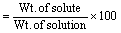Example: 10%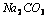solution w/w means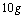ofis dissolved in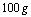of the solution. (It meansis dissolved in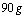of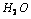)

(ii) Weight to volume percent

% w/v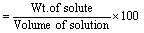Example: 10%(w/v) meansis dissolved in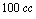of solution.

(iii) Volume to volume percent

% v/v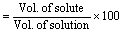Example:10% ethanol (v/v) means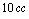of ethanol dissolved in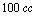of solution.

(iv) Volume to weight percent

% v/w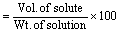Example: 10% ethanol (v/w) means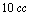of ethanol dissolved inof solution.

(2) Parts per million (ppm) and parts per billion (ppb) : When a solute is present in trace quantities, it is convenient to express the concentration in parts per million and parts per billion. It is the number of parts of solute per million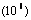or per billion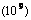parts of the solution. It is independent of the temperature.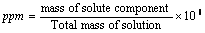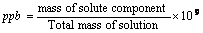(3) Strength: The strength of solution is defined as the amount of solute in grams present in one litre (or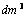) of the solution. It is expressed in g/litre or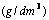.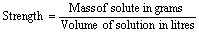(4) Normality (N) : It is defined as the number of gram equivalents (equivalent weight in grams) of a solute present per litre of the solution. Unit of normality is gram equivalents litre–1. Normality changes with temperature since it involves volume. When a solution is diluted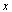times, its normality also decreases by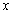times. Solutions in term of normality generally expressed as,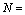Normal solution;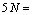Penta normal,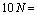Deca normal;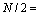semi normal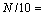Deci normal;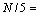Penti normal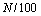or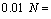centinormal,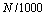or 0.001= millinormal

Mathematically normality can be calculated by following formulas,

(i)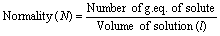(ii) N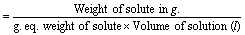(iii)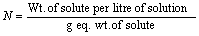(iv)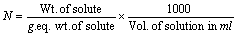(v)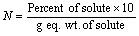,

(vi)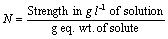(vii)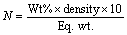(viii) If volume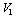and normality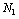is so changed that new normality and volume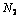and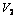then,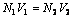(Normality equation)

(ix) When two solutions of the same solute are mixed then normality of mixture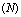is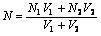(x) Vol. of water to be added i.e.,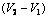to get a solution of normalityfrom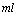of normality(xi) If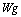of an acid is completely neutralised by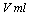of base of normality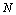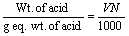Similarly,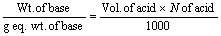(xii) When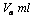of acid of normality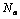is mixed with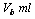of base of normality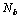(a) If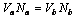(Solution neutral)

(b) If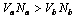(Solution is acidic)

(c) If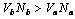(Solution is basic)

(xiii) Normality of the acidic mixture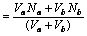(xiv) Normality of the basic mixture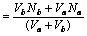(xv)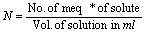(* 1 equivalent = 1000 milliequivalent or meq.)

## NEET & AIIMS Exam Sample Papers

 AIIMS SAMPLE PAPERS View More NEET SAMPLE PAPERS View More